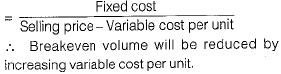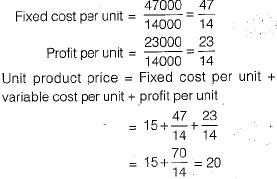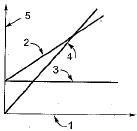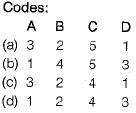Courses

# Test: Break Even Analysis - 2

## 7 Questions MCQ Test Topicwise Question Bank for Mechanical Engineering | Test: Break Even Analysis - 2

Description
This mock test of Test: Break Even Analysis - 2 for Mechanical Engineering helps you for every Mechanical Engineering entrance exam. This contains 7 Multiple Choice Questions for Mechanical Engineering Test: Break Even Analysis - 2 (mcq) to study with solutions a complete question bank. The solved questions answers in this Test: Break Even Analysis - 2 quiz give you a good mix of easy questions and tough questions. Mechanical Engineering students definitely take this Test: Break Even Analysis - 2 exercise for a better result in the exam. You can find other Test: Break Even Analysis - 2 extra questions, long questions & short questions for Mechanical Engineering on EduRev as well by searching above.
QUESTION: 1

Solution:
QUESTION: 2

Solution:
QUESTION: 3

### The breakeven volume for a product can be reduced by

Solution:

Breakeven volumeQUESTION: 4

A company sells 14,000 units of its product. It has a variable cost of Rs. 15 per unit. Fixed cost is Rs. 47,000 and total required profit is Rs. 23,000. Per unit product price (in Rs.) will be

Solution:

Total number of product sold by a company =14000
Variable cost per unit = Rs. 15
Fixed cost = Rs. 47,000
Total profit = Rs. 23,000QUESTION: 5

Match List-I (Cost/revenue parameter) with List-ll (Break-even chart’s parameter) and select the correct answer using the code given below the lists:
List-I
A. Facility cost
B. Total cost
C. Sales revenue
D. Production quantity
List-lISolution:
QUESTION: 6

Consider the following statements in respect of break-even point:
1. Revenue is equal to total cost.
2. Revenue is equal to variable cost.
3. Profit/Loss is equal to zero.
Which of these statements is/are correct?

Solution:
QUESTION: 7

If a company’s total sales is Rs. 50,000 and (P/V) ratio is 50% and margin of safety percentage is 40%, then break-even point sale is

Solution: